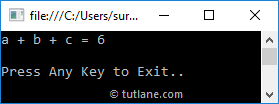In c#, Method Overloading means defining a multiple methods with same name but with different parameters. By using Method Overloading, we can perform a different tasks with same method name by passing different parameters.

Suppose, if we want to overload a method in c#, then we need to define another method with same name but with different signatures. In c#, the Method Overloading is also called as compile time polymorphism or early binding.

Following is the code snippet of implementing a method overloading in c# programming language.

public class Calculate

{

public void AddNumbers(int a, int b)

{

Console.WriteLine("a + b = {0}", a + b);

}

public void AddNumbers(int a, int b, int c)

{

Console.WriteLine("a + b + c = {0}", a + b + c);

}

}

If you observe above “Calculate” class, we defined a two methods with same name (AddNumbers) but with different input parameters to achieve method overloading in c#.

Following is the example of implementing a method overloading in c# programming language.

using System;

namespace Tutlane

{

public class Calculate

{

public void AddNumbers(int a, int b)

{

Console.WriteLine("a + b = {0}", a + b);

}

public void AddNumbers(int a, int b, int c)

{

Console.WriteLine("a + b + c = {0}", a + b + c);

}

}

class Program

{

static void Main(string[] args)

{

Calculate c = new Calculate();

Console.WriteLine("\nPress Enter Key to Exit..");

}

}

}

When we execute above c# program, we will get the result like as shown below.This is how we can implement method overloading in c# by defining a multiple methods with same name but with different signatures based on our requirements.